GeeksforGeeks App
Open AppBrowser
Continue

# C Program for Red Black Tree Insertion

Following article is extension of article discussed here.
In AVL tree insertion, we used rotation as a tool to do balancing after insertion caused imbalance. In Red-Black tree, we use two tools to do balancing.

1. Recoloring
2. Rotation

We try recoloring first, if recoloring doesn’t work, then we go for rotation. Following is detailed algorithm. The algorithms has mainly two cases depending upon the color of uncle. If uncle is red, we do recoloring. If uncle is black, we do rotations and/or recoloring.
Color of a NULL node is considered as BLACK.

Let x be the newly inserted node.

1. Perform standard BST insertion and make the color of newly inserted nodes as RED.
2. If x is root, change color of x as BLACK (Black height of complete tree increases by 1).
3. Do following if color of x’s parent is not BLACK or x is not root.
1. If x’s uncle is RED (Grand parent must have been black from property 4
1. Change color of parent and uncle as BLACK.
2. color of grand parent as RED.
3.     3. Change x = x’s grandparent, repeat steps 2 and 3 for new x.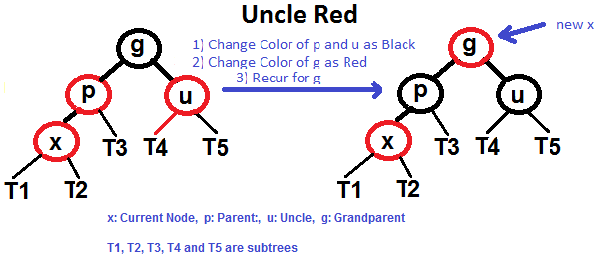2. If x’s uncle is BLACK, then there can be four configurations for x, x’s parent (p) and x’s grandparent (g) (This is similar to AVL Tree

1. Determine the configuration:
1. Left Case (p is left child of g and x is left child of p).
2. Left Right Case (p is left child of g and x is right child of p).
3. Right Right Case (Mirror of case a).
4. Right Left Case (Mirror of case c).
2. Change x = x’s parent, repeat steps 2 and 3 for new x.

Following are operations to be performed in four subcases when uncle is BLACK.

## All four cases when Uncle is BLACK

Left Left Case (See g, p and x)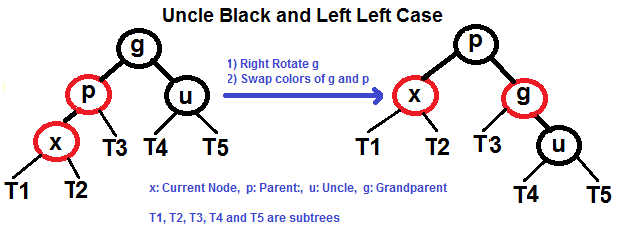Left Right Case (See g, p and x)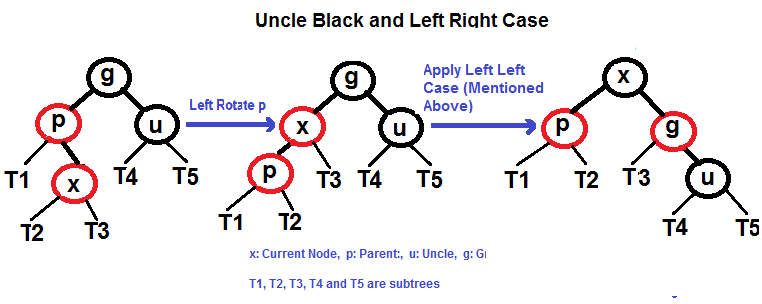Right Right Case (See g, p and x)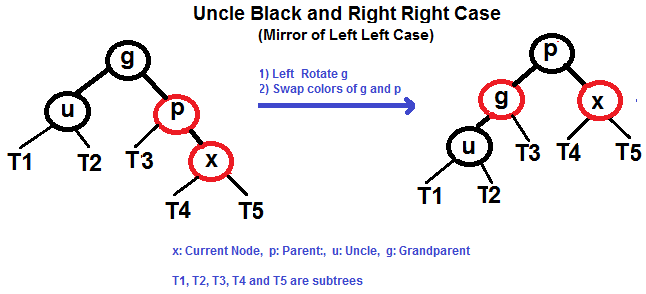Right Left Case (See g, p and x)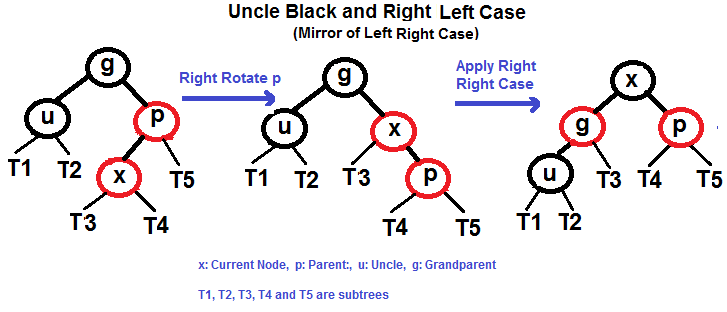Examples of Insertion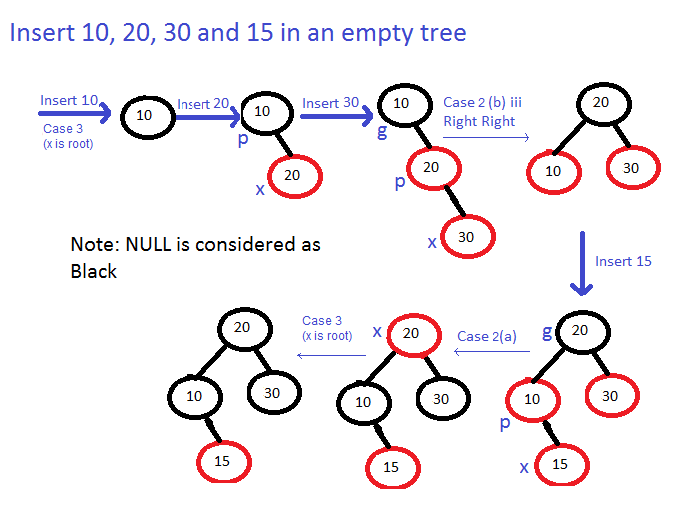Implementation:

## C

 `/** C implementation for``    ``Red-Black Tree Insertion``    ``This code is provided by``    ``costheta_z **/``#include ``#include ` `// Structure to represent each``// node in a red-black tree``struct` `node {``    ``int` `d; ``// data``    ``int` `c; ``// 1-red, 0-black``    ``struct` `node* p; ``// parent``    ``struct` `node* r; ``// right-child``    ``struct` `node* l; ``// left child``};` `// global root for the entire tree``struct` `node* root = NULL;` `// function to perform BST insertion of a node``struct` `node* bst(``struct` `node* trav,``                      ``struct` `node* temp)``{``    ``// If the tree is empty,``    ``// return a new node``    ``if` `(trav == NULL)``        ``return` `temp;` `    ``// Otherwise recur down the tree``    ``if` `(temp->d < trav->d)``    ``{``        ``trav->l = bst(trav->l, temp);``        ``trav->l->p = trav;``    ``}``    ``else` `if` `(temp->d > trav->d)``    ``{``        ``trav->r = bst(trav->r, temp);``        ``trav->r->p = trav;``    ``}` `    ``// Return the (unchanged) node pointer``    ``return` `trav;``}` `// Function performing right rotation``// of the passed node``void` `rightrotate(``struct` `node* temp)``{``    ``struct` `node* left = temp->l;``    ``temp->l = left->r;``    ``if` `(temp->l)``        ``temp->l->p = temp;``    ``left->p = temp->p;``    ``if` `(!temp->p)``        ``root = left;``    ``else` `if` `(temp == temp->p->l)``        ``temp->p->l = left;``    ``else``        ``temp->p->r = left;``    ``left->r = temp;``    ``temp->p = left;``}` `// Function performing left rotation``// of the passed node``void` `leftrotate(``struct` `node* temp)``{``    ``struct` `node* right = temp->r;``    ``temp->r = right->l;``    ``if` `(temp->r)``        ``temp->r->p = temp;``    ``right->p = temp->p;``    ``if` `(!temp->p)``        ``root = right;``    ``else` `if` `(temp == temp->p->l)``        ``temp->p->l = right;``    ``else``        ``temp->p->r = right;``    ``right->l = temp;``    ``temp->p = right;``}` `// This function fixes violations``// caused by BST insertion``void` `fixup(``struct` `node* root, ``struct` `node* pt)``{``    ``struct` `node* parent_pt = NULL;``    ``struct` `node* grand_parent_pt = NULL;` `    ``while` `((pt != root) && (pt->c != 0)``           ``&& (pt->p->c == 1))``    ``{``        ``parent_pt = pt->p;``        ``grand_parent_pt = pt->p->p;` `        ``/*  Case : A``             ``Parent of pt is left child``             ``of Grand-parent of``           ``pt */``        ``if` `(parent_pt == grand_parent_pt->l)``        ``{` `            ``struct` `node* uncle_pt = grand_parent_pt->r;` `            ``/* Case : 1``                ``The uncle of pt is also red``                ``Only Recoloring required */``            ``if` `(uncle_pt != NULL && uncle_pt->c == 1)``            ``{``                ``grand_parent_pt->c = 1;``                ``parent_pt->c = 0;``                ``uncle_pt->c = 0;``                ``pt = grand_parent_pt;``            ``}` `            ``else` `{` `                ``/* Case : 2``                     ``pt is right child of its parent``                     ``Left-rotation required */``                ``if` `(pt == parent_pt->r) {``                    ``leftrotate(parent_pt);``                    ``pt = parent_pt;``                    ``parent_pt = pt->p;``                ``}` `                ``/* Case : 3``                     ``pt is left child of its parent``                     ``Right-rotation required */``                ``rightrotate(grand_parent_pt);``                ``int` `t = parent_pt->c;``                ``parent_pt->c = grand_parent_pt->c;``                ``grand_parent_pt->c = t;``                ``pt = parent_pt;``            ``}``        ``}` `        ``/* Case : B``             ``Parent of pt is right``             ``child of Grand-parent of``           ``pt */``        ``else` `{``            ``struct` `node* uncle_pt = grand_parent_pt->l;` `            ``/*  Case : 1``                ``The uncle of pt is also red``                ``Only Recoloring required */``            ``if` `((uncle_pt != NULL) && (uncle_pt->c == 1))``            ``{``                ``grand_parent_pt->c = 1;``                ``parent_pt->c = 0;``                ``uncle_pt->c = 0;``                ``pt = grand_parent_pt;``            ``}``            ``else` `{``                ``/* Case : 2``                   ``pt is left child of its parent``                   ``Right-rotation required */``                ``if` `(pt == parent_pt->l) {``                    ``rightrotate(parent_pt);``                    ``pt = parent_pt;``                    ``parent_pt = pt->p;``                ``}` `                ``/* Case : 3``                     ``pt is right child of its parent``                     ``Left-rotation required */``                ``leftrotate(grand_parent_pt);``                ``int` `t = parent_pt->c;``                ``parent_pt->c = grand_parent_pt->c;``                ``grand_parent_pt->c = t;``                ``pt = parent_pt;``            ``}``        ``}``    ``}``}` `// Function to print inorder traversal``// of the fixated tree``void` `inorder(``struct` `node* trav)``{``    ``if` `(trav == NULL)``        ``return``;``    ``inorder(trav->l);``    ``printf``(``"%d "``, trav->d);``    ``inorder(trav->r);``}` `// driver code``int` `main()``{``    ``int` `n = 7;``    ``int` `a = { 7, 6, 5, 4, 3, 2, 1 };` `    ``for` `(``int` `i = 0; i < n; i++) {` `        ``// allocating memory to the node and initializing:``        ``// 1. color as red``        ``// 2. parent, left and right pointers as NULL``        ``// 3. data as i-th value in the array``        ``struct` `node* temp``            ``= (``struct` `node*)``malloc``(``sizeof``(``struct` `node));``        ``temp->r = NULL;``        ``temp->l = NULL;``        ``temp->p = NULL;``        ``temp->d = a[i];``        ``temp->c = 1;` `        ``// calling function that performs bst insertion of``        ``// this newly created node``        ``root = bst(root, temp);` `        ``// calling function to preserve properties of rb``        ``// tree``        ``fixup(root, temp);``          ``root->c = 0;``    ``}` `    ``printf``(``"Inorder Traversal of Created Tree\n"``);``    ``inorder(root);` `    ``return` `0;``}`

Output

```Inorder Traversal of Created Tree
1  2  3  4  5  6  7

Level Order Traversal of Created Tree
6  4  7  2  5  1  3  ```

Time Complexity:  O(log n), as the height of red-black tree is O(log n) at most, and complexity of rotation is constant.
Auxiliary Space:  O(n),  here ‘n’ is the number of nodes in the red-black trees.

My Personal Notes arrow_drop_up